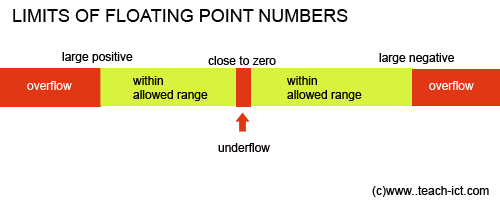# 9. Going beyond the limit

If a calculation exceeds the limits of the floating point scheme then the system is designed to flag an error in the CPU. A good program will take note of this error warning and do something about it (other than simply crashing in a heap!)

If the range goes beyond the most positive or most negative largest number, this is called an 'overflow'. Trying to divide a number by zero is certain to cause an overflow.

If the number is too tiny to be represented, this is called an 'underflow'. For example dividing a number by a very large number may cause an underflow.

### Overflow and Underflow### Truncation

Some numbers have infinte decimal points (irrational numbers) for example 1/3 as a decimal is 0.333 recurring.

But floating point can only represent so many decimal digits. Therefore 'Truncation' will occur.

Truncation is where decimal values beyond the truncation point are simply discarded and it causes errors in floating point calculations.

### Rounding

If you have a number such as 3.456 then if you have to round this to three significant digits, then the number becomes 3.46 and a small error called the 'rounding error' has occured

Note that the CPU will not flag any error when truncation and rounding occurs, as it is actiing within its limits. All a programmer can do is carefully assess whether his calculations will lead to significant rounding and truncation errors.

`     `